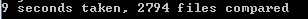## Go Recursive Diff to Verify SVN Merge December 16, 2014

For some reason, SVN Merge over large trees, where work was simultaneously going on in both branches, is very unreliable. And, you can't tell quickly if something is wrong, especially if what is different is not compiled. This could be very bad if you merge your current work into the trunk, and deploy the trunk version live. It would require a full regression test.

Fortunately, Go exists, and is fast. However, with all of my tweaking of goroutintes and channels to try to get it to process each subdirectory in a separate goroutine, my efforts proved futile as I couldn't get it to be quicker than 9 seconds. There's a lot of content. I wrote it to ignore whitespace changes so that slowed it down immensely.

`package utility`

```import (     "fmt"     "crypto/md5" )```

```func MD5(content []byte) string {     sum := md5.Sum(content)     return fmt.Sprintf("%x", sum) }```

That's the utility class that I use to just hash large swaths of content. Then here's the huge chunk of code that is my recursive file diff, approximately 170 lines of code.

`package main`

```import (     "io/ioutil"     "os"     "fmt"     "strings"     "utility"     "regexp"     "time" )```

```type Dir struct {     Name string     FullPath string     BaseDir *Dir     Subdirs []*Dir     Files []string     Level int }```

```type FileResult struct {     FullPath string     Result bool }```

`var reg = regexp.MustCompile(`[\W]+`)`

```func readDirRecursive(base *Dir, types, ignore []string) {     content, err := ioutil.ReadDir(base.FullPath)```

```    if err != nil {         return     }     for _, f := range content {         name := f.Name()         if f.IsDir() {             addDir := true             for _, ign := range ignore {                 addDir = addDir && !strings.EqualFold(name, ign)             }```

```            if addDir {                 sub := &Dir{ Name: name, BaseDir: base, FullPath: base.FullPath + `\` + name, Level: base.Level + 1}                 readDirRecursive(sub, types, ignore)                 base.Subdirs = append(base.Subdirs, sub)             }         } else {             addFile := false             for _, t := range types {                 addFile = addFile || strings.HasSuffix(name, t)             }             if addFile {                 base.Files = append(base.Files, name)             }         }     } }```

```func spaces(times int) string{     return strings.Repeat(" ", times) }```

```func printDir (level int, dir *Dir){     fmt.Print(spaces(level) + dir.Name + "\n")     for _, sd := range dir.Subdirs {         printDir(level +1, sd)     }```

```    for _, f := range dir.Files {         fmt.Println(spaces(level) + "- " + f)     } }```

```func getContentMD5(file string) string {     b,err := ioutil.ReadFile(file)     if err != nil {         fmt.Println(err)         return nil     }```

```    s := reg.ReplaceAllString(string(b), "")     return utility.MD5([]byte(s)) }```

```func compareFiles(file1, file2 string) bool {     m1 := getContentMD5(file1)     m2 := getContentMD5(file2)         return m1 == m2 }```

```func compinternal (dir1 *Dir, dir2 *Dir, results chan FileResult) {     for _, f := range dir1.Files {         for _, f2 := range dir2.Files {             if strings.EqualFold(f,f2) {                 result := compareFiles(dir1.FullPath + `\` + f, dir2.FullPath + `\` + f2)                 results <- FileResult{ FullPath: dir1.FullPath + `\` + f, Result: result}                 break             }         }     }```

```    for _, sd1 := range dir1.Subdirs {         for _, sd2 := range dir2.Subdirs {             if strings.EqualFold(sd1.Name, sd2.Name){                 sdchan := make(chan FileResult)                 go func(){                     compinternal(sd1, sd2, sdchan)                     close(sdchan)                 }()```

```                for sdresult := range sdchan {                     results <- sdresult                 }```

```                break             }         }     } }```

```func rcomp(dir1, dir2 string, filetypes []string, ignore []string) []string {     diffs := []string{}```

```    left := &Dir{ Name: "Root Left", FullPath: dir1, Level: 0, BaseDir: nil }     right := &Dir{ Name: "Root Right", FullPath: dir2, Level: 0, BaseDir: nil }```

```    readDirRecursive(left, filetypes, ignore)     readDirRecursive(right, filetypes, ignore)```

```    resultChannel := make(chan FileResult)     go func (){          compinternal(left, right, resultChannel)         close(resultChannel)     }()```

```    for result := range resultChannel {         if !result.Result {             diffs = append(diffs, result.FullPath)         }     }```

```    return diffs }```

```func main(){     args := os.Args[1:]```

```    if len(args) < 4 {         fmt.Println("need right and left directories, file types to include, folder names to ignore")         return     }```

`    start := time.Now().Unix()`

```    types := strings.Split(args, ";")     ignore := strings.Split(args, ";")```

```    fmt.Println("Grabbing files " + strings.Join(types, ", "))     fmt.Println("Ignoring folders " + strings.Join(ignore, ", "))```

```    diffs := rcomp(args,args, types, ignore)     for _, diff := range diffs {         fmt.Println(diff)     }```

`    end := time.Now().Unix()`

`    total := end - start`

```    fmt.Println(total, "seconds taken") }```I later added a counter to see how many files it's actually comparing. In the project I wrote this app for, the file count of those included (.js, .cs, etc) was 2,794.I could stand to clean up the output a bit, but it helped me identify a few files that were out of date with the branch. Thanks svn. And thanks Go!

2021
2020
2019
2018
2017
2016
2015
2014
2013
2012
2011
2010
2009
2008
2007
2006
2005